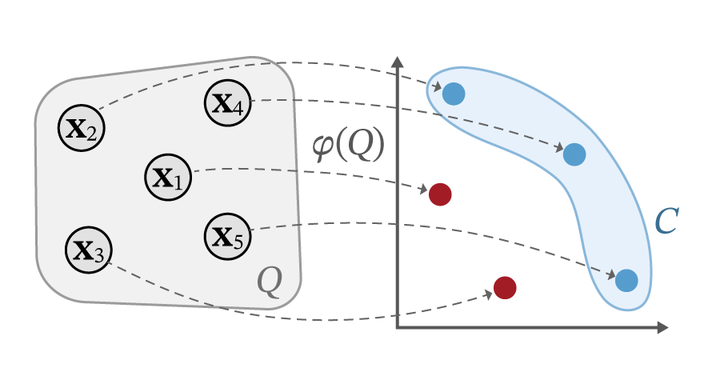# Learning Choice Functions via Pareto-Embeddings### Abstract

We consider the problem of learning to choose from a given set of objects, where each object is represented by a feature vector. Traditional approaches in choice modelling are mainly based on learning a latent, real-valued utility function, thereby inducing a linear order on choice alternatives. While this approach is suitable for discrete (top-1) choices, it is not straightforward how to use it for subset choices. Instead of mapping choice alternatives to the real number line, we propose to embed them into a higher-dimensional utility space, in which we identify choice sets with Pareto-optimal points. To this end, we propose a learning algorithm that minimizes a differentiable loss function suitable for this task. We demonstrate the feasibility of learning a Pareto-embedding on a suite of benchmark datasets.

Date
2020-09-22 17:00 — 18:30
Location
Bamberg, Germany

# Virtual Poster

## IntroductionA person choosing out of a set of headphones.We consider a choice problem where a decision maker is confronted with a set of alternatives of which they have to choose a subset. Our ultimate goal is to explain and predict the observed choices. Every alternative is represented by a vector of features. A popular strategy in choice modelling is to assume that the choice probabilities depend on an underlying (latent) real-valued utility function of the decision maker. Under this assumption, learning comes down to identifying the parameters of such a utility function. This works well for the case where only single alternatives are chosen, but is non-trivial to apply to our setting where subsets of alternatives are chosen. Either one faces combinatorial problems calculating the probabilities for many subsets, or has to resort to thresholding techniques. It would be desirable to have an approach which is able to learn a utility function and the choices result naturally given this function.

## Research Questions

• Can we generalize learning of a utility function that admits a natural choice function?
• Are we able to learn such a function from data?

## Pareto-Embeddings

Let $\mathcal{X} \subseteq \mathbb{R}^d$ be the set of objects. A set of objects $Q = {\mathbf{x}_1, \dots, \mathbf{x}_m}$ we call a query. A classical approach to model choices is to assume the existence of a utility function $\mathcal{X} \to \mathbb{R}$. A singular choice then arises naturally as picking the object with highest utility.A Pareto-embedding $\varphi$ maps a given set of objects into a higher-dimensional space $\mathcal{Z}$.To solve the problem of learning subset choices, we propose to embed the objects into a special higher-dimensional utility space $\mathcal{Z} \subseteq \mathbb{R}^{d’}$, in which subset choices are naturally identified by Pareto-optimal points (see Figure 2). A point $\mathbf{z}_i$ in the embedding space is dominated by another point $\mathbf{z}_j$ if $z_{i, k} \leq z_{j, k}$ for all $k \in [d’]$ and $z_{i, k} < z_{j, k}$ for at least one such $k$. Then, a point $\mathbf{z}_i$ is called Pareto-optimal (with respect to $Z$), if it is not dominated by any other point $\mathbf{z}_j \in Z$, $1 \leq j\neq i \leq m$.

To induce a suitable embedding function $\varphi$ from data, we devise a differentiable loss function tailored to this task (see the paper for more details). The loss function consists of multiple terms of which the following two are the most important.$L_{\text{PO}}$ penalizes all objects that dominate a chosen objects.With the first loss term $L_{\text{PO}}$, depicted in Figure 3, we ensure that all objects which have been chosen, are not dominated in the new space $\mathcal{Z}$.$L_{\text{DOM}}$ penalizes the object closest to dominating an unchosen object.Now for all objects which were not chosen from the input set, we only have to ensure that one object dominates it. The second loss term $L_{\text{DOM}}$, depicted in Figure 4, does so by determining the object which is closest to dominating the unchosen object and penalizing it.

## ResultsEach object is passed through a (deep) multi-layer perceptron followed by a linear output layer to produce the embedding.We utilize the loss function as part of a deep learning pipeline to investigate the feasibility of learning such a Pareto-embedding (see Figure 5).

We evaluate our approach on a suite of benchmark problems from the field of multi-criteria optimization. We use the well-known DTLZ and ZDT test suites, containing datasets of varying difficulty. Adding a simple two-dimensional two parabola (TP) dataset, we end up with 14 benchmark problems in total.Results of the empirical evaluation. The bars show the average performance in terms of A-mean across the 5 outer splits. The sticks show the estimated standard deviation.

The results are shown in Figure 6. For five of the problems, the embedding approach is able to achieve an average A-mean of over 90%, indicating that for these problems we often identify the choice set correctly. This is important, as it shows that the loss function is able to steer the model parameters towards a valid Pareto-embedding. Thus, it is apparent that our learner is performing better than random guessing on all datasets.

## Conclusion

• We proposed a novel way to tackle the problem of learning choice functions as an embedding problem.
• To learn an embedding from given data, we develop a suitable loss function that penalizes violations of the Pareto condition.
• The first results on benchmark problems are promising and we are looking forward to a more extensive empirical evaluation and applications to real-world choice problems.